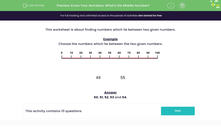# Find The Middle Numbers

In this worksheet, students will select the numbers that go between two given numbers.Key stage:  KS 1

Curriculum topic:   Number: Number and Place Value

Curriculum subtopic:   Order/Compare Numbers to 100

Popular topics:   Numbers worksheets

Difficulty level:#### Worksheet Overview

This activity is about finding numbers that lie between two given numbers.

Example

Choose the numbers that lie between the two given numbers.49 55

If we look at the number line above, we can see where 49 is - it is the red line just before the number 50. We need to count each number that comes after 49 until we reach the number 55. The first number after 49 is 50, so that's where we start: 50, 51, 52, 53, 54.

50, 51, 52, 53 and 54.

Note that it asked for the numbers between 49 and 55. This means that we can't include the numbers 49 or 55 themselves.

Shall we have a go at some questions now?### What is EdPlace?

We're your National Curriculum aligned online education content provider helping each child succeed in English, maths and science from year 1 to GCSE. With an EdPlace account you’ll be able to track and measure progress, helping each child achieve their best. We build confidence and attainment by personalising each child’s learning at a level that suits them.

Get started